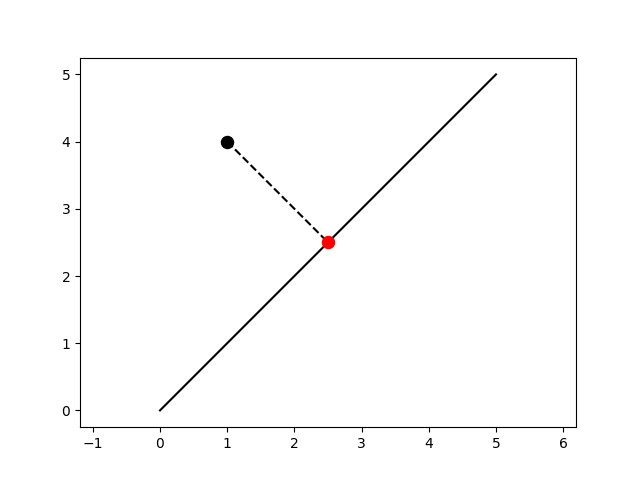# 2D Point-Line Projection¶

Project a point onto a line.Out:

```(-0.25, 5.25, -0.25, 5.25)
```

```from skspatial.objects import Line, Point
from skspatial.plotting import plot_2d

line = Line(point=[0, 0], direction=[1, 1])
point = Point([1, 4])

point_projected = line.project_point(point)
line_projection = Line.from_points(point, point_projected)

_, ax = plot_2d(
line.plotter(t_2=5, c='k'),
line_projection.plotter(c='k', linestyle='--'),
point.plotter(s=75, c='k'),
point_projected.plotter(c='r', s=75, zorder=3),
)

ax.axis('equal')
```

Total running time of the script: ( 0 minutes 0.119 seconds)

Gallery generated by Sphinx-Gallery